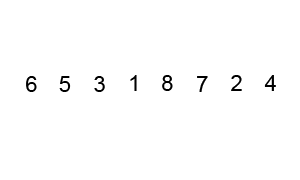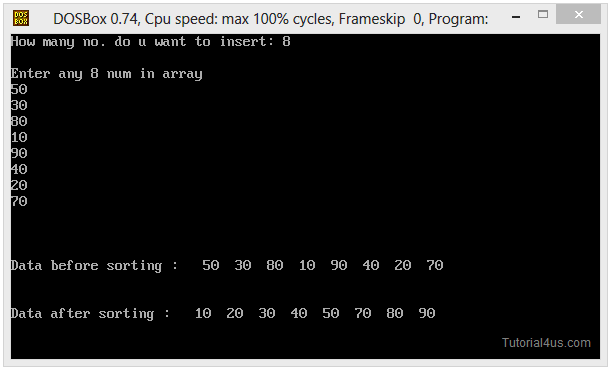# Bubble Sort in C++

## Bubble Sort Program in C++

Bubble sort is the simplest sorting algorithm. In this technique we follow given step to short given elements in increasing order.

### Steps to Sort data

• First compare First (previous) element with its next elements.
• If next element is grater than previous element just ignore it.
• If next element is smaller than previous element then interchange their position.### Advantage of Bubble short in C++

It is very simple and easy method to short elements.

### Dis-Advantage of Bubble short in C++

It is time comsuming and slow process to short elements.

## Bubble Sort Program in C++

```
#include<iostream.h>
#include<conio.h>
#include<dos.h>

void main()
{
int i,a,temp,j,no;
clrscr();
cout<<"How many no. do u want to insert: ";
cin>>no;
cout<<"\nEnter any"<<no<<"num in array \n";

for(i=0;i<no;i++)
{
cin>>a[i];
}
cout<<"\n\n\nData before sorting : ";
for(j=0;j<no;j++)
{
delay(200);
cout<<"  "<<a[j];
}
for(i=0;i<no;i++)
{
for(j=0;j<no-i-1;j++)
{
if(a[j]>a[j+1])
{
temp=a[j];
a[j]=a[j+1];
a[j+1]=temp;
}
}
}
cout<<"\n\n\nData after sorting : ";
for(j=0;j<no;j++)
{
delay(200);
cout<<"   "<<a[j];
}
getch();
}
```

## Output

```
How many no. do u want to insert: 8
Enter any 8  num in array
Data before sorting : 5 3 6 2 7 8 1 4
Data after sorting : 1 2 3 4 5 6 7 8
```